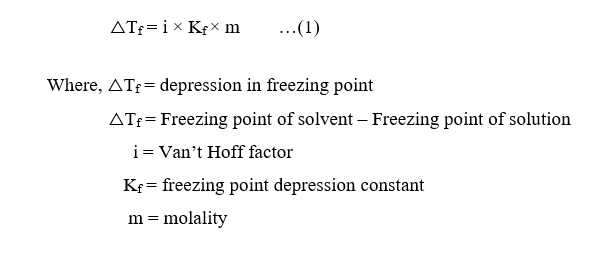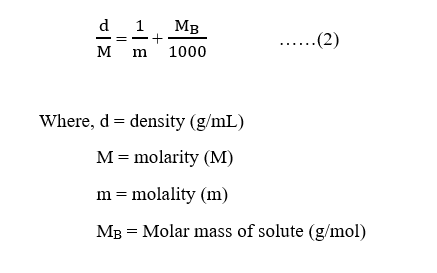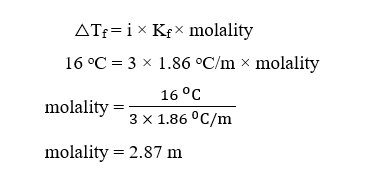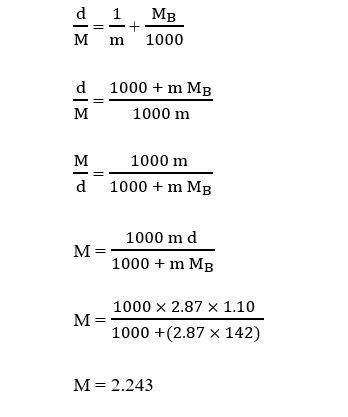# An aqueous solution of Na2SO4 is prepared and its measured freezing point is -16.0o C. Calculate the molarity of the solution. The density of the solution is 1.10g/mL. Kf for water is 1.86o C/m.

Question
50 views

An aqueous solution of Na2SOis prepared and its measured freezing point is -16.0o C. Calculate the molarity of the solution. The density of the solution is 1.10g/mL. Kfor water is 1.86o C/m.

check_circle

Step 1

The depression or lowering in freezing point is expressed as:The molality  and molarity are related as follows :Step 2

Given,

Freezing point of solution, Tf = -16.0 oC

Freezing point of solvent (water), Tfo = 0 oC

Freezing point depression constant, Kf = 1.86 oC/m

Density of the solution = 1.10g/mL

Na2SO4 (aq) →2  Na+ (aq) + SO42- (aq)

Here, i = number of ions dissociated = 2 + 1 = 3

And,

△Tf = Freezing point of solvent – Freezing point of solution

△Tf = 0 oC – (–16.0 oC)

△Tf = 16.0 oC

Substituting the values of △Tf , i and Kf in equation (1), we get,Step 3

Now, given density, d =  1.10g/mL

Molar mass of Na2SO4 , MB = (2×23) + 32 + (4×16) = 142 g/mol

The molarity can be calculated using equation (2) as follows :...

### Want to see the full answer?

See Solution

#### Want to see this answer and more?

Solutions are written by subject experts who are available 24/7. Questions are typically answered within 1 hour.*

See Solution
*Response times may vary by subject and question.
Tagged in

### Chemistry